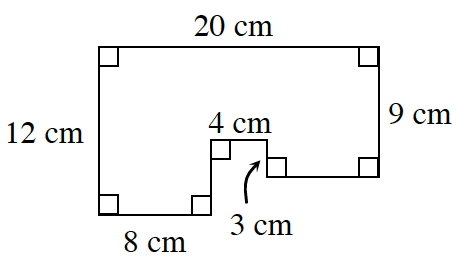### Home > INT2 > Chapter 8 > Lesson 8.2.2 > Problem8-46

8-46.

At right is a scale drawing of the floor plan for Nzinga’s dollhouse. The actual dimensions of the dollhouse are five times the measurements provided in the floor plan at right.

1. Use the measurements provided in the diagram to calculate the area and perimeter of her floor plan.

Divide the diagram into three separate rectangles.
Calculate the area of each individual rectangle, then add them all together.
Then calculate the perimeter.1. Draw a similar figure on your paper. Label the sides with the actual measurements of Nzinga’s dollhouse. What is the perimeter and area of the floor of her actual dollhouse? Show all work.

When you draw the similar figure, be sure to multiply each of the side lengths by $5$. Then use the same process you did in part (a) to calculate the are and perimeter.

2. What is the ratio of the perimeters of the two figures? What do you notice?

3. What is the ratio of the areas of the two figures? How does the ratio of the areas seem to be related to the scale factor? Explain.